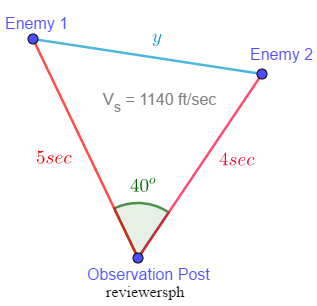### Math Notes

Subjects

#### Trigonometry Solutions

##### Topics || Problems

A military observer notes two enemy batteries which subtend, at his observation post, an angle of $$40^o$$. The interval between the flash and the report of a gun is 5 seconds for one battery, and 4 seconds for the other. If the velocity of sound is 1140 ft a second, how far apart are the batteries?To calculate (approximate) the distance between the enemies, calculate first the distance between the army post to the enemies.

The distance between the post and enemy 1 can be approximated by using the speed of sound and the time interval between the flash and the report. Thus, $$d_1 = \frac{1140 ~ft}{sec} (5 ~sec) = 5700 ~ft$$

Same with enemy 2, $$d_2 = \frac{1140 ~ft}{~sec} (4) = 4560 ~ft$$

Calculate the distance between them.

$$y^2 = 5700^2 + 4560^2 - 2 (4560)(5700) \cos 40$$

The two enemies are probably $$y = 3669 ~ft$$ apart. Answer

Will you be doing trigonometry in the heat of a fight?